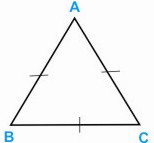HOME MATH DICTIONARY DOWNLOAD FEEDBACK DISCLAIMER
 Question: What is an Equilateral Triangle ? Answer: An equilateral triangle is a triangle whose sides are all of equal length. Its angles are all 60°. In geometry, an equilateral triangle is a triangle in which all three sides have equal lengths. In traditional or Euclidean geometry, equilateral triangles are also equiangular; that is, all three internal angles are also equal to each other and are each 60°. They are regular polygons, and can therefore also be referred to as regular triangles. An equilateral triangle can be easily constructed using a compass. Example of an Equilateral Triangle: